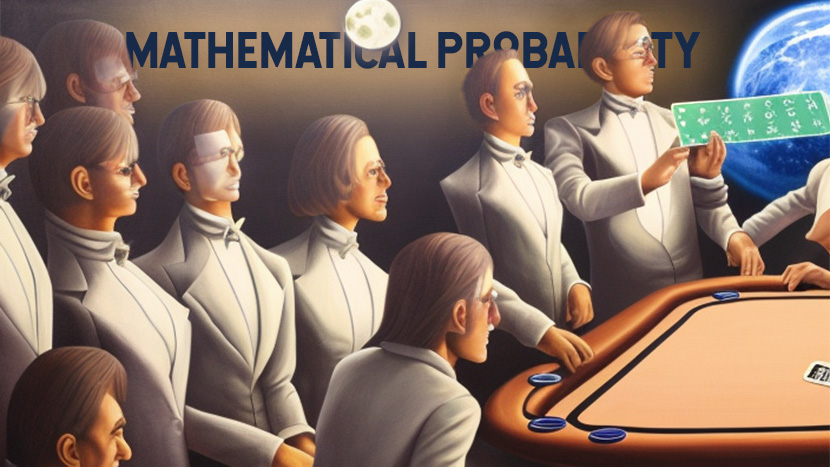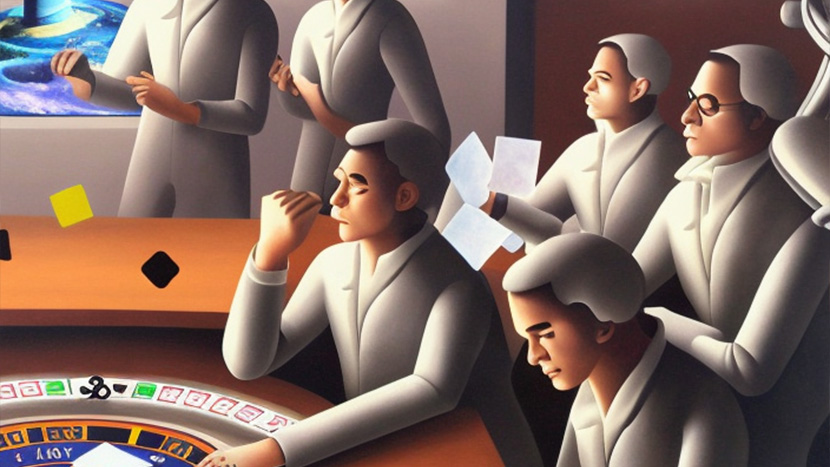# Understanding The Mathematical Probability Behind The Game Of Blackjack

Blackjack is a card game loved by many, and it has a lot of mathematical probability embedded into it. Just like humans digestive system has a set way of working, so does probability in blackjack.In this article, we are going to discuss mathematical probability, its connection to blackjack, and how we can help you better understand it so that you may improve your blackjack skills and construct a strategy for success

# The Basics

First, with regard to the games that are played with full decks of cards in a casino, we will discuss the probabilities that are related with the dealing of the cards and the first predictions.

Let’s have a look at the odds of getting a good starting hand (the first two cards that are given to you). For a game played with one deck, the total number of potential combinations for each of the two cards is C(52, 2) = 1326, whereas for a game played with two decks, this number is C(104, 2) = 5356.

In a game with one deck, the probability of getting a natural blackjack is P = 8/663 = 1.20663%, whereas in a game with two decks, the probability of getting a natural blackjack is P = 16/1339 = 1.19492%.

In a game with one deck, the probability of getting a blackjack with the first two cards is calculated as P = 32/663 = 4.82654%, but in a game with two decks, the probability is calculated as P = 64/1339 = 4.77968%.

# Probability of Points

In a game with one deck, the chance of obtaining 20 points from the first two cards is P = 68/663 = 10.25641%, whereas in a game with two decks, the probability of getting 20 points from the first two cards is P = 140/1339 = 10.45556%.

In a game with one deck, the probability of earning 19 points from the first two cards is P = 40/663 = 6.03318%, but in a game with two decks, the probability of obtaining 19 points from the first two cards is P = 80/1339 = 5.97460%.

In a game with one deck, the probability of getting 18 points from the first two cards is P = 43/663 = 6.48567%, whereas in a game with two decks, the probability of getting 18 points from the first two cards is P = 87/1339 = 6.4973%.

In a game with one deck, the probability of receiving 17 points from the first two cards is P = 16/221 = 7.23981%, whereas in a game with two decks, the probability of earning 17 points from the first two cards is P = 96/1339 = 7.16952%.

A blackjack or a hand with 20, 19, or 18 points is an example of a good starting hand (one with which you may remain) in blackjack. P = 32/663 + 68/663 + 40/663 + 43/663 = 183/663, in the case of a 1-deck game, and P = 64/1339 + 140/1339 + 80/1339 + 87/1339 = 371/1339, in the case of a 2-deck game. The probability of getting such a hand is calculated by adding up all of the probabilities that correspond to it: P = 32/663 + 68/663 + 40/663 + 43/6

In a game with one deck, the probability of getting a strong starting hand is calculated as P = 183/663 = 27.60180%, but in a game with two decks, the probability is calculated as P = 371/1339 = 27.70724%.### Written by Austin Crane

Austin is the principle web director for Untamed Science and Stone Age Man. He is also the web-director of the series for the High School biology, Middle Grades Science and Elementary Science content. When Austin isn't making amazing content for the web, he's out on his mountain bike or in a canoe.